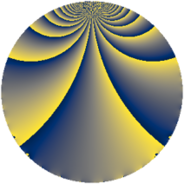# Properties

 Label 552.2.nLevel $552$ Weight $2$ Character orbit 552.n Rep. character $\chi_{552}(91,\cdot)$ Character field $\Q$ Dimension $48$ Newform subspaces $2$ Sturm bound $192$ Trace bound $2$

# Related objects

## Defining parameters

 Level: $$N$$ $$=$$ $$552 = 2^{3} \cdot 3 \cdot 23$$ Weight: $$k$$ $$=$$ $$2$$ Character orbit: $$[\chi]$$ $$=$$ 552.n (of order $$2$$ and degree $$1$$) Character conductor: $$\operatorname{cond}(\chi)$$ $$=$$ $$184$$ Character field: $$\Q$$ Newform subspaces: $$2$$ Sturm bound: $$192$$ Trace bound: $$2$$ Distinguishing $$T_p$$: $$5$$

## Dimensions

The following table gives the dimensions of various subspaces of $$M_{2}(552, [\chi])$$.

Total New Old
Modular forms 100 48 52
Cusp forms 92 48 44
Eisenstein series 8 0 8

## Trace form

 $$48q - 4q^{2} + 8q^{4} - 4q^{6} - 4q^{8} + 48q^{9} + O(q^{10})$$ $$48q - 4q^{2} + 8q^{4} - 4q^{6} - 4q^{8} + 48q^{9} + 8q^{16} - 4q^{18} - 4q^{24} + 48q^{25} - 4q^{32} + 8q^{36} - 40q^{46} + 48q^{49} - 84q^{50} - 4q^{54} - 80q^{62} + 8q^{64} - 4q^{72} + 48q^{81} - 80q^{82} - 12q^{92} - 8q^{94} - 4q^{96} - 20q^{98} + O(q^{100})$$

## Decomposition of $$S_{2}^{\mathrm{new}}(552, [\chi])$$ into newform subspaces

Label Dim. $$A$$ Field CM Traces $q$-expansion
$$a_2$$ $$a_3$$ $$a_5$$ $$a_7$$
552.2.n.a $$24$$ $$4.408$$ None $$-4$$ $$24$$ $$0$$ $$0$$
552.2.n.b $$24$$ $$4.408$$ None $$0$$ $$-24$$ $$0$$ $$0$$

## Decomposition of $$S_{2}^{\mathrm{old}}(552, [\chi])$$ into lower level spaces

$$S_{2}^{\mathrm{old}}(552, [\chi]) \cong$$ $$S_{2}^{\mathrm{new}}(184, [\chi])$$$$^{\oplus 2}$$